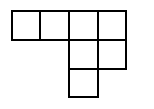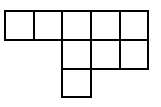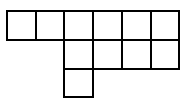### Home > AC > Chapter 5 > Lesson 5.1.5 > Problem5-52

5-52.

Find the rule for the following tile pattern. 2-59 HW eTool (Desmos)  Homework Help ✎Figure 2 Figure 3 Figure 4

What is the growth rate? How many tiles is each figure increasing by?
(This is the slope, or the $m$ value)

What is the starting point? How many tiles does Figure 0 have?
(This is the $y$-intercept, or the $b$ value)

Use this information to create a $y=mx+b$ equation that represents the pattern.

Use the table in the eTool below to graph the rule for the tile pattern.
Click the link at right for the full version of the eTool: CCA 2-59 HW eTool